Flatten

The basic use of Flatten is demonstrated in the next cell.  Lots of users know about the basic use of Flatten, but there is much more to the story.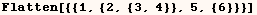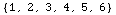First of all Flatten will flatten nested layers of any head as demonsrated in the next cell.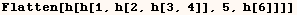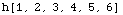When Flatten is given only one argument, it checks the head of the expression and flattens nested layers of that head.  Notice the nested lists in the next example aren't flattened.  Also in  this example we are flattening layers of (h), starting with the arguments of (expr) and going down to deeper levels.  When Flatten gets to a subexpression that doesn't have the head (h) it decides not to look inside this subexpression for nested layers of (h).  Hence the output has nested layers of (h) in {h[h,h]}.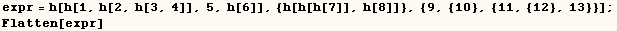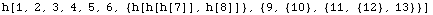In the next input we give Flatten (2) as a second argument and only two layers of (h) are flattened.  Here too the subexpression g[h[8,h[9,10]]] doesn't have the head (h), so Flatten doesn't flatten layers of (h) in this subexpression.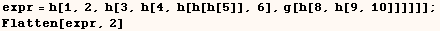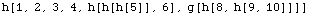We can give Flatten a third argument to indicate that layers with a certain head should be flattened. This is needed in the next cell to ensure layers of (h) are flattened.  If Flatten was given one or two arguments it would try to flatten layers of (g).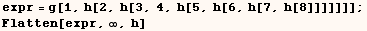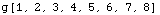In the next example Flatten is trying to flatten layers of (h).  Flatten finds that the subexpression g[h[9,h[10,11]]] doesn't have the head (h) so it doesn't flatten layers of (h) in that subexpression.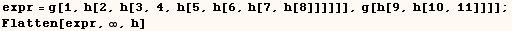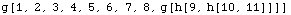If we want to flatten all nested layers of (h), the replacement rule in the next cell should be used.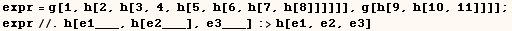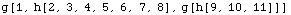Created by Mathematica  (May 16, 2004)

Back to Ted’s Tricks index page